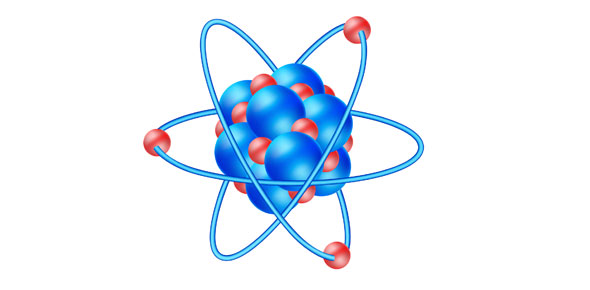# Atomic Structure & Periodic Table Test Quiz

10 Questions | Total Attempts: 40SettingsThis quiz will test your on your fundamental knowledge on the atomic structure. Get ready! oh, and you will need your periodic table.

• 1.
Carbon-12 and Carbon-14 are _______________.
• A.

Ions

• B.

Isotopes

• C.

Different elements

• D.

Compounds

• 2.
The atom oxygen has a neucleon number of 16 and a proton number of 8. what is the number of neutrons in the oxygen atom?
• A.

4

• B.

16

• C.

8

• D.

0

• 3.
Hydrogen, deuterium and tritium are all isotopes of hydrogen. They have a different number of _____________.
• A.

Atoms

• B.

Neutrons

• C.

Protons

• D.

Electrons

• 4.
Element P has a nucleon number of 31 and a proton number of 15. Eement S has a nucleon number of 32 and a proton number of 16. The two elements have the same __________.
• A.

Number of protons

• B.

Number of neutrons

• C.

Nucleon number

• D.

Number of electrons

• 5.
Vanadium, V has a nucleon number of 51 and a proton number of 23. how many electrons does it have?
• A.

51

• B.

79

• C.

28

• D.

23

• 6.
Hydrogen has a nucleon number of 1 and a proton number of 1. how many neutrons does deuterium, an isotope of hydrogen, have?
• A.

0

• B.

3

• C.

2

• D.

1

• 7.
Element X has a nucleon number of 40 and a proton number of 20. Which period in the periodic table is X found in?
• A.

Period 2

• B.

Period 1

• C.

Period 7

• D.

Period 5

• 8.
How many electrons does an isotope of  Oxygen, nucleon number 16 and proton number 8, have?
• A.

8

• B.

6

• C.

10

• D.

2

• 9.
“Isotopes are elements that have the same number of neutrons and different number of protons.” What is wrong with the previous sentence?
• A.

Isotopes are elements that have the same number of protons and different number of neutrons.

• B.

Isotopes are elements that have the same number of protons and different number of electrons.

• C.

Isotopes are elements that have the same number of neutrons and different number of electrons.

• D.

Isotopes are elements that have the same number of electrons and different number of protons.

• 10.
A beam of particles containing protons, neutrons and electrons is fired through an electric field. Which particle will not bend or deviate?
• A.

Proton

• B.

Electron

• C.

Neutron

• D.

All of the above

Related TopicsBack to top